# 技术让梦想更伟大资料

ID：技术让梦想更伟大

## 了解一下类型转换

``int a = 9; float b = 8.5; ``

a，b占的字节大小不一样，这个我们应该都知道，在C语言中一个表达式允许不同类型的数据进行运算，例如:

``int a = 9; float b = 8.5，c; c = a + b; ``

## 自动类型转换

``//vs2019 //来源：技术让梦想更伟大 //作者：李肖遥 #include <stdio.h> int main() {  //定义一个整型指针变量pPoint  int* pPoint;   //定义基本的数据的类型  char c;  short s;  int i;  long l;  float f;  double d;   //将整型浮点型数据赋值给指针类型  pPoint = c;  pPoint = s;  pPoint = i;  pPoint = l;  pPoint = f;  pPoint = d;    return 0; } ``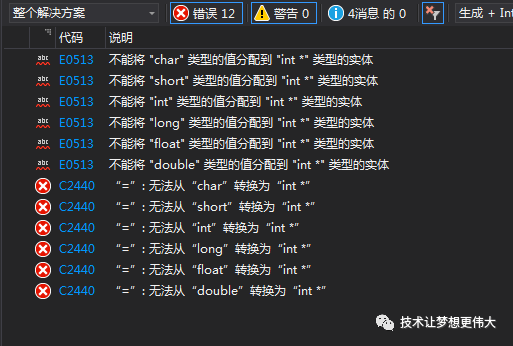``//vs2019 //来源：技术让梦想更伟大 //作者：李肖遥 #include <stdio.h> int main() {  //定义一个整型指针变量pPoint  int* pPoint;   //定义基本的数据的类型  char c;  short s;  int i;  long l;  float f;  double d;   //将整型浮点型数据运算之后赋值给指针类型  pPoint = c + c;  pPoint = s + s;  pPoint = i + i;  pPoint = l + l;  pPoint = f + f;  pPoint = d + d;    return 0; } ``
• char同类型运算，结果是一个int类型。
• short同类型运算，结果是一个int类型。
• int同类型运算，结果是一个int类型。
• long同类型运算，结果是一个long类型。
• float同类型运算，结果是一个float类型。
• double同类型运算，结果是一个double类型。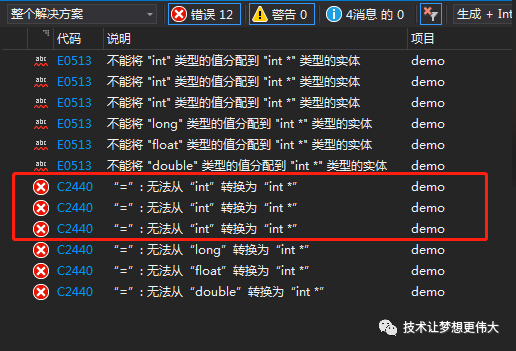• 整型：比int小的，都会转换成int，比int大的不变。
• 浮点：不变。

``//vs2019 //来源：技术让梦想更伟大 //作者：李肖遥 #include <stdio.h> int main() {  //定义一个整型指针变量pPoint  int* pPoint;   //定义基本的数据的类型  char c;  short s;  int i;  long l;  float f;  double d;   //将整型浮点型数据混合运算赋值给指针类型  pPoint = c + s;  // char + short = int  pPoint = c + i;  // char + int = int  pPoint = c + l;  // char + long = int  pPoint = c + f;  // char + float = long  pPoint = c + d;  // char + double = float    return 0; } ``
• char类型与short类型运算，结果是一个int类型。
• char类型与int类型运算，结果是一个int类型。
• char类型与long类型运算，结果是一个long类型。
• char类型与float类型运算，结果是一个float类型。
• char类型与double类型运算，结果是一个double类型。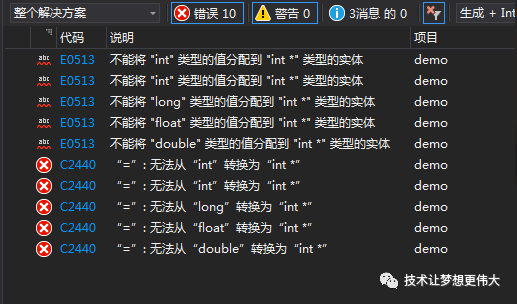• 如果两边均比int小或等于int，那么结果为int。
• 如果两边有比int大的，那么结果为比int大的类型。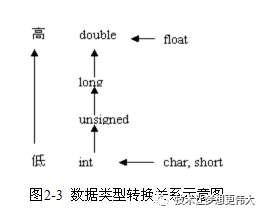• 整型类型级别从低到高依次为：
int -> unsigned int -> long -> unsigned long -> long long -> unsigned long long

• 浮点型级别从低到高依次为：
float -> double

• 图中横向箭头表示必须的转换，如两个float型数参加运算，虽然它们类型相同，但仍要先转成double型再进行运算，结果亦为double型。
• 图中纵向箭头表示当运算符两边的运算数为不同类型时的转换，如一个long 型数据与一个int型数据一起运算，需要先将int型数据转换为long型， 然后两者再进行运算，结果为long型。
• 当较高类型的数据转换为较低类型时，则可能有些数据丢失。
• 当较低类型的数据转换为较高类型时，一般只是形式上有所改变， 而不影响数据的实质内容。
• 所有这些转换都是由系统自动进行的，使用时你只需从中了解结果的类型即可。

## 强制类型转换

``（类型说明符） (表达式) ``

``//vs2019 //来源：技术让梦想更伟大 //作者：李肖遥 #include<stdio.h> #include<string.h>  int main() {     float f,x=1.3,y=1.4;     int i = 4,a,b;     a = x + y;     b = (int)(x+y);     f = 10/i;     printf("a=%d,b=%d,f=%f,x=%f,y=%fn",a,b,f,x,y); } ``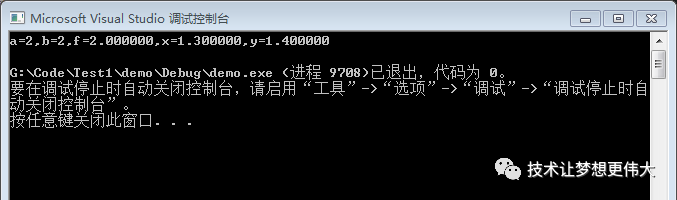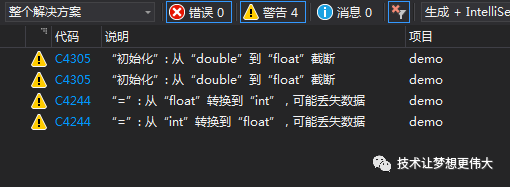1. 这个数据类型表示的内存空间的大小。
2. 编译器把设定的数值放到这个内存空间，是数据类型的存储方式解析后存进去的。

## 总结强调一点

qklbishe.com区块链毕设代做网专注|以太坊fabric-计算机|java|毕业设计|代做平台 » 技术让梦想更伟大资料